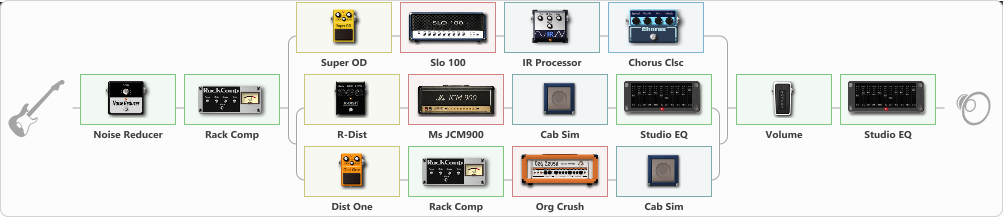# Black Album tone (Mid edition)

Discussion in 'ToneLib-GFX presets' started by clever1, Jun 15, 2021.

1. Black Album tone (Mid edition)

Preset name: Black Album

This is preset is the Mid range focused version of the Black album tone. If you are recording, these should be atleast 2 tracks panned to 35 Right/35 Left and a little lower than the Mid Scooped guitars. Note: The video has the Mid focused preset, along with the Mid scooped preset.

Related video:

Effects chain:Effect: "Noise Reducer" (Dynamics / Filter), active - "yes"
{
"Sens" = 75
"Mode" = Soft
}

Effect: "Rack Comp" (Dynamics / Filter), active - "yes"
{
"Threshold (dB)" = -20
"Ratio" = 5
"Attack" = Fast
"Release (ms)" = 250
"Knee" = 25
"Level (dB)" = 0
}

Effect: "Splitter" (Dynamics / Filter)
{
"A-Bypass" = Off
"A-Pan" = 31
"A-Level" = 58
"B-Bypass" = Off
"B-Pan" = -33
"B-Level" = 49

'A' branch:
{

Effect: "Super OD" (Overdrive / Distortion), active - "yes"
{
"Drive" = 57
"Tone" = 66
"Level" = 91
}

Effect: "Slo 100" (Amp simulators), active - "yes"
{
"Gain" = 56
"Bass" = 86
"Middle" = 74
"Treble" = 60
"Presence" = 75
"Master" = 68
"Level (dB)" = 2
}

Effect: "IR Processor" (Cabinets), active - "yes"
{
"IR" = Black_L
"Low Cut (Hz)" = 0
"Hi Cut (kHz)" = 20.0
"Mix" = 100
"Level (dB)" = 0
}

Effect: "Chorus Clsc" (Modulation / Sfx), active - "yes"
{
"Speed" = 2.0
"Depth" = 47
"Center" = 1.7
"Mode" = Stereo
}
}
'B' branch:
{

Effect: "Splitter" (Dynamics / Filter)
{
"A-Bypass" = Off
"A-Pan" = -50
"A-Level" = 59
"B-Bypass" = Off
"B-Pan" = 62
"B-Level" = 51

'A' branch:
{

Effect: "R-Dist" (Overdrive / Distortion), active - "yes"
{
"Drive" = 58
"Tone" = 28
"Level" = 50
}

Effect: "Ms JCM900" (Amp simulators), active - "yes"
{
"Gain" = 69
"Bass" = 63
"Middle" = 37
"Treble" = 58
"Presence" = 62
"Master" = 73
"Level (dB)" = 0
}

Effect: "Cab Sim" (Cabinets), active - "yes"
{
"Model" = 4x12" 1960 T75
"Level (dB)" = 0
}

Effect: "Studio EQ" (Dynamics / Filter), active - "yes"
{
"31 Hz" = 0
"62 Hz" = -2
"125 Hz" = 0
"250 Hz" = 0
"500 Hz" = 2
"1 kHz" = 1
"2 kHz" = 1
"4 kHz" = -3
"8 kHz" = -3
"16 kHz" = 0
"Level (dB)" = 0
}
}
'B' branch:
{

Effect: "Dist One" (Overdrive / Distortion), active - "yes"
{
"Drive" = 29
"Tone" = 59
"Level" = 37
}

Effect: "Rack Comp" (Dynamics / Filter), active - "yes"
{
"Threshold (dB)" = -20
"Ratio" = 5
"Attack" = Fast
"Release (ms)" = 250
"Knee" = 25
"Level (dB)" = 0
}

Effect: "Org Crush" (Amp simulators), active - "yes"
{
"Gain" = 69
"Bass" = 59
"Middle" = 23
"Treble" = 34
"Presence" = 66
"Master" = 65
"Level (dB)" = 0
}

Effect: "Cab Sim" (Cabinets), active - "yes"
{
"Model" = 4x12" Cali v30
"Level (dB)" = 0
}
}
}
}
}

Effect: "Volume" (Dynamics / Filter), active - "yes"
{
"Pedal Pos." = 51
"Pan" = 0
}

Effect: "Studio EQ" (Dynamics / Filter), active - "yes"
{
"31 Hz" = 2
"62 Hz" = 0
"125 Hz" = 1
"250 Hz" = 1
"500 Hz" = -1
"1 kHz" = -2
"2 kHz" = -2
"4 kHz" = 2
"8 kHz" = 1
"16 kHz" = 1
"Level (dB)" = 0
}

Note: You will need to download and install the ToneLib-GFX software to use the preset.

File size:
2.5 KB
Views:
2,707